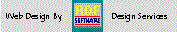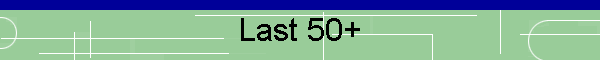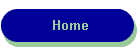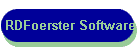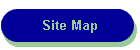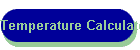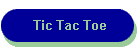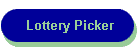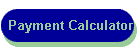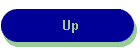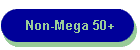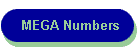RDF-Home

LUGIE
Linux Users Group

Zenbot CNC
User's Group

The first Super Lotto Plus game {#1375} was drawn on June 7th. 2000.  The winning numbers from the most recent games are shown below.  To play this NEW game, you still pick 6 numbers, but choose 5 numbers in the range of 1 to 47 and the Mega {or 6th} number in the range of 1 to 27.  There are 9 ways to win with this new game, so be sure to check the rules at the California Lottery site.

The chart below shows the frequency of numbers selected from the most recent Pick-6 Super Lottery Drawings: Game #3104  thru 3050  Updated 01/05/17

Magenta = Mega Number Range
Black = Additional Numbers Pick-5 range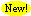Check out Non-Mega Number frequencies here!

Check out Mega Number frequencies here!

Last Week's Game Results:

 Game 3104 1    3    6  10  46  47 12/31/16 Game 3103 6  10  13  16  34  41 12/28/16
`** Meg`
```
Number Frequency for the California State Lottery

Num=  1 Count=  10    Num= 25 Count=   9

Num=  2 Count=  10    Num= 26 Count=   4

Num=  3 Count=  10    Num= 27 Count=   9

Num=  4 Count=   5    Num= 28 Count=   6

Num=  5 Count=   6    Num= 29 Count=   6

Num=  6 Count=  10    Num= 30 Count=   8

Num=  7 Count=   5    Num= 31 Count=   7

Num=  8 Count=   7    Num= 32 Count=   5

Num=  9 Count=   4    Num= 33 Count=   4

Num= 10 Count=  10    Num= 34 Count=   7

Num= 11 Count=   7    Num= 35 Count=   5

Num= 12 Count=   7    Num= 36 Count=   6

Num= 13 Count=   7    Num= 37 Count=   9

Num= 14 Count=   3    Num= 38 Count=   3

Num= 15 Count=  12    Num= 39 Count=  10

Num= 16 Count=   9    Num= 40 Count=   7

Num= 17 Count=   7    Num= 41 Count=   6

Num= 18 Count=   9    Num= 42 Count=   4

Num= 19 Count=   4    Num= 43 Count=   6

Num= 20 Count=   7    Num= 44 Count=   8

Num= 21 Count=   8    Num= 45 Count=   5

Num= 22 Count=   5    Num= 46 Count=   8

Num= 23 Count=   8    Num= 47 Count=   7

Num= 24 Count=  11

Valid Numbers in this series=330 from 55 most recent Games

Frequency Range:  Max=12 Min=3

Most Frequent Numbers:

Number   15 Frequency=  12

Target Frequency:   8

Number   21 Frequency=   8

Number   23 Frequency=   8

Number   30 Frequency=   8

Number   44 Frequency=   8

Number   46 Frequency=   8

Least Frequent Numbers:

Number    9 Frequency=   4

Number   19 Frequency=   4

Number   26 Frequency=   4

Number   33 Frequency=   4

Number   42 Frequency=   4

Number   14 Frequency=   3

Number   38 Frequency=   3

```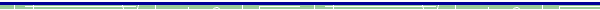dBase, Delphi  and C++ Builder  are trademarks of Borland International, Inc. All other products mentioned are registered trademarks or trademarks of their respective companies. Questions or problems regarding this web site should be directed to RDF@RDFoerster.com. Copyright © 1997-2007 RDFoerster Software. All rights reserved. Last modified: Thursday January 05, 2017